# ORELA Middle Grades Math: Exponents & Exponential Expressions Chapter Exam

Exam Instructions:

Choose your answers to the questions and click 'Next' to see the next set of questions. You can skip questions if you would like and come back to them later with the yellow "Go To First Skipped Question" button. When you have completed the practice exam, a green submit button will appear. Click it to see your results. Good luck!

### Page 1

#### Question 1 1. Simplify the following expression using positive exponents.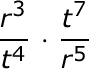#### Question 3 3. Simplify using ONLY positive exponents.#### Question 4 4. Solve the following expression: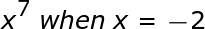#### Question 5 5. Which of the following is equivalent to the expression below?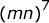### Page 2

#### Question 7 7. Simplify the following expression using positive exponents only.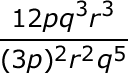#### Question 8 8. Which of the following is equivalent to the expression below?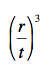#### Question 9 9. Simplify the following expression.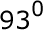#### Question 10 10. Simplify the expression below.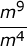### Page 3

#### Question 11 11. Which of the following exponential expressions is equal to this radical expression?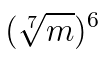#### Question 12 12. Which of the following is equivalent to the following expression?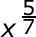#### Question 13 13. Which of the following is equivalent to the expression below?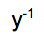#### Question 14 14. Which term corresponds to the exponent?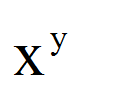#### Question 15 15. Simplify the expression using positive exponents.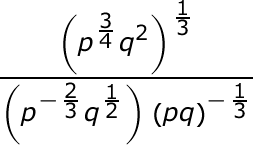### Page 4

#### Question 17 17. Which of the following is equivalent to the expression below?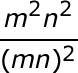#### Question 18 18. Which of the following is equivalent to the following expression?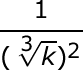#### Question 19 19. Which of the following would be true regarding the following exponential expression?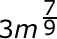#### Question 20 20. Which number is the base?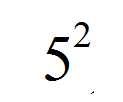### Page 5

#### Question 22 22. Which of the following radical expressions is equal to this exponential expression?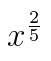#### Question 23 23. Simplify the following expression: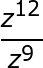#### Question 24 24. Simplify using only positive exponents.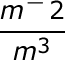#### Question 25 25. Simplify the expression using positive exponents.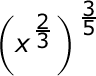### Page 6

#### Question 26 26. Simplify the expression below using only positive exponents.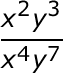#### Question 27 27. Simplify the expression below using positive exponents only.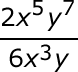#### Question 29 29. Simplify the expression using positive exponents.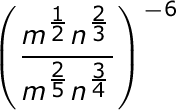#### ORELA Middle Grades Math: Exponents & Exponential Expressions Chapter Exam Instructions

Choose your answers to the questions and click 'Next' to see the next set of questions. You can skip questions if you would like and come back to them later with the yellow "Go To First Skipped Question" button. When you have completed the practice exam, a green submit button will appear. Click it to see your results. Good luck!

Support